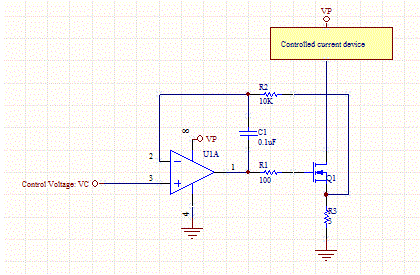# Voltage Controlled Current Source - Current Servo

This circuit allows a current device to be controlled with a small control voltage. The op-amp loop forces the output current to be proportional to the control voltage VC.The current to the controlled device is equal to the current through R3, the current sense resistor. The currents through R1 and R2 are essentially zero, so we can neglect their voltage drops in the circuit. The op-amp's negative feedback will adjust the current through the MOSFET until the voltage across R3 is equal to the control voltage. Thus, the voltage across R3 is equal to the control voltage VC.

IR3=VC/R3

The control voltage should be limited such that the maximum current of the controlled device is not exceeded. Typically, the voltage is a few hundred millivolts. C1 provides some low pass filtering of the feedback.

[Plant Database], [Soil Moisture Sensor] [Water Level Sensor] [Soil Moisture Meter]